# Creating a BEM volume conduction model of the head for source-reconstruction of EEG data

## Introduction

In this tutorial you can find information about how to construct a Boundary Element Method (BEM) volume conduction model of the head (head model) based on a single subject’s MRI. We will use the anatomical images that belong to the same subject whose data was analyzed in other tutorials. The anatomical MRI data is included in the Subject01.zip MEG dataset.

The volume conduction model shown here is EEG specific. In reality, we do not have corresponding EEG data to the anatomical MRI we use in this tutorial, but we will use a template EEG set to demonstrate how to build a head model for EEG and how to align the electrodes to anatomical data. Different strategies can be used for the construction of head models. The processing pipeline of the tutorial is an example which we think is the most appropriate for the tutorial-dataset.

This tutorial will not show how to perform the source reconstruction itself. If you are interested in source reconstruction methods, you can go to the Localizing oscillatory sources using beamformer techniques and to the Source reconstruction of event-related fields using minimum-norm estimate tutorials.

Furthermore, if you are interested in MEG head models, you can go to the corresponding MEG tutorial.

## Background

The EEG/MEG signals measured on the scalp do not directly reflect the location of the activated neurons. To reconstruct the location and the time-course or spectral content of a source in the brain, various source-localization methods are available. You can read more about the different methods in review papers suggested here.

The level of the activity at a source location is estimated from

1. the EEG/MEG activity measured on or around the scalp
2. the spatial arrangement of the electrodes/gradiometers (sensor positions),
3. the geometrical and conductive properties of the head (head model)
4. the location of the source (source model)

Using this information, source estimation comprises two major steps: (1) Estimation of the potential or field distribution for a known source and for a known model of the head is referred to as forward modeling. (2) Estimation of the unknown sources corresponding to the measured EEG or MEG is referred to as inverse modeling.

The forward solution can be computed when the head model, the sensor positions and the source is given. For distributed source models and for scanning approaches (such as beamforming), the source model is discretizing the brain volume into a volumetric or surface grid. When the forward solution is computed, the lead field matrix (= channels X source points matrix) is calculated for each grid point taking into account the head model and the sensor positions.

A prerequisite of forward modeling is that the geometrical description of all elements (sensor positions, head model and source model) is registered in the same coordination system with the same units. There are different “conventions” how to define a coordinate system, but the precise coordinate system is not relevant, as long as all data is expressed in it consistently (i.e. in the same coordinate system). Here read more about how the different head and mri coordinate systems are defined. The MEG sensors by default are defined relative to anatomical landmarks of the head (the fiducial coils), therefore when the anatomical images are also aligned to these landmarks, the MEG sensors do not need to be re-aligned. EEG data is typically not aligned to the head, therefore, the electrodes have to be re-aligned prior to source-reconstruction (see also this faq and this example).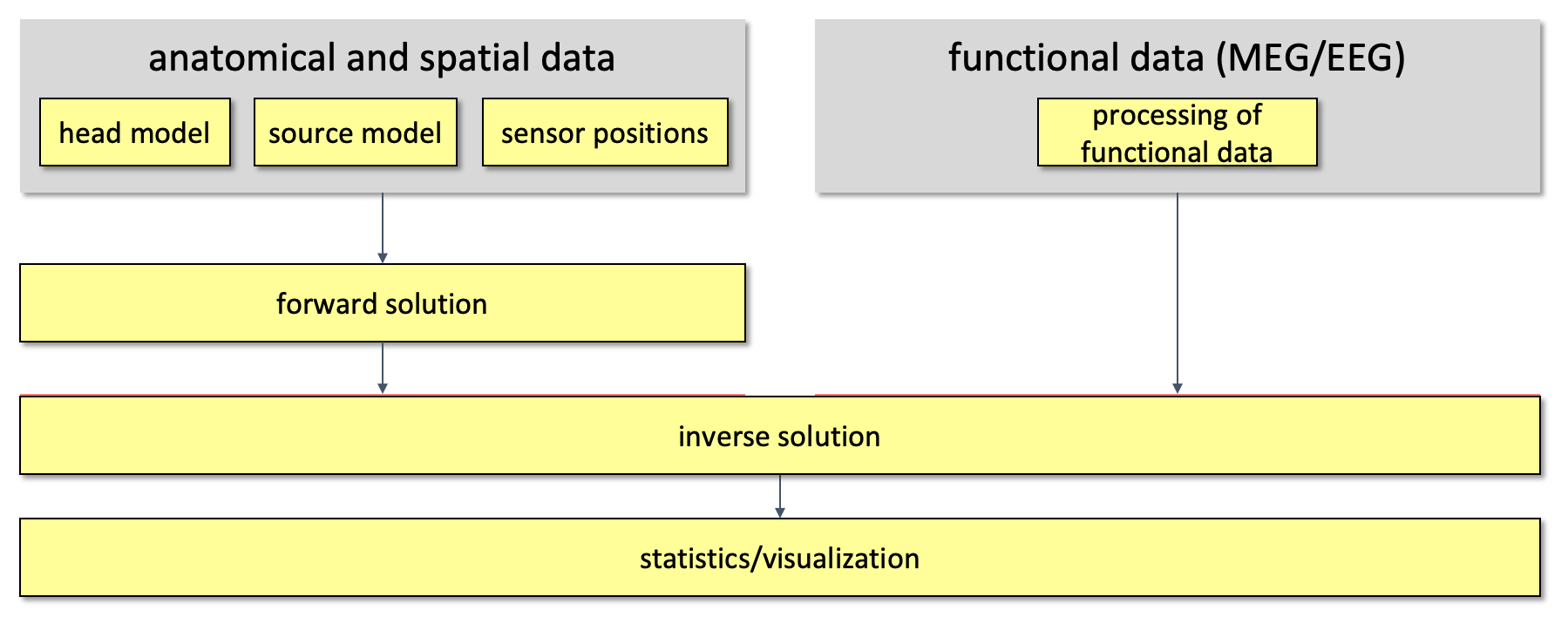Figure 1. Major steps in source reconstruction

This tutorial is focusing on how to build the volume conduction model for the head.

In FieldTrip a volume conduction model is represented as a MATLAB structure which is usually indicated with the variable name vol. It describes how the currents flow through the tissue, not where they originate from. In general it consists of a description of the geometry of the head, a description of the conductivity of the tissue, and mathematical parameters that are derived from these. Whether and how the mathematical parameters are described depends on the computational solution to the forward problem either by numerical approximations, such as the boundary element and finite element method (BEM and FEM), or by exact analytical solutions (e.g., for spherical models).

The more accurate the description of the geometry of the head or the source, the better the quality of the forward model. There are many types of head models which, to various degrees, take the individual anatomy into account. The different head models available in FieldTrip are listed here.

If an anatomical MRI is not available for your EEG subject, you can consider to use a template MRI or a template head model that is located in the FieldTrip template directory. See here for more info.

If you do not have an MRI, but do have a measurement of the scalp surface or electrodes (e.g., with a Polhemus tracker), you can fit a concentric spheres volume conduction model to the scalp.

## Procedure

Here, we will work towards a volume conduction model of the head based on the boundary element method (BEM). The BEM model assumes realistic information (of a certain degree) about the interface between the skin, skull and brain surfaces. First, we will use an anatomical MRI to extract these surfaces. This procedure is termed segmentation. Following the segmentation, a description of each surface using vertices and triangles is constructed. Finally, the BEM model will be computed.

The anatomical mri of the tutorial data set is available here. Although we did not record EEG in this particular language study, we will nevertheless use it as example MRI to make an EEG volume conduction model.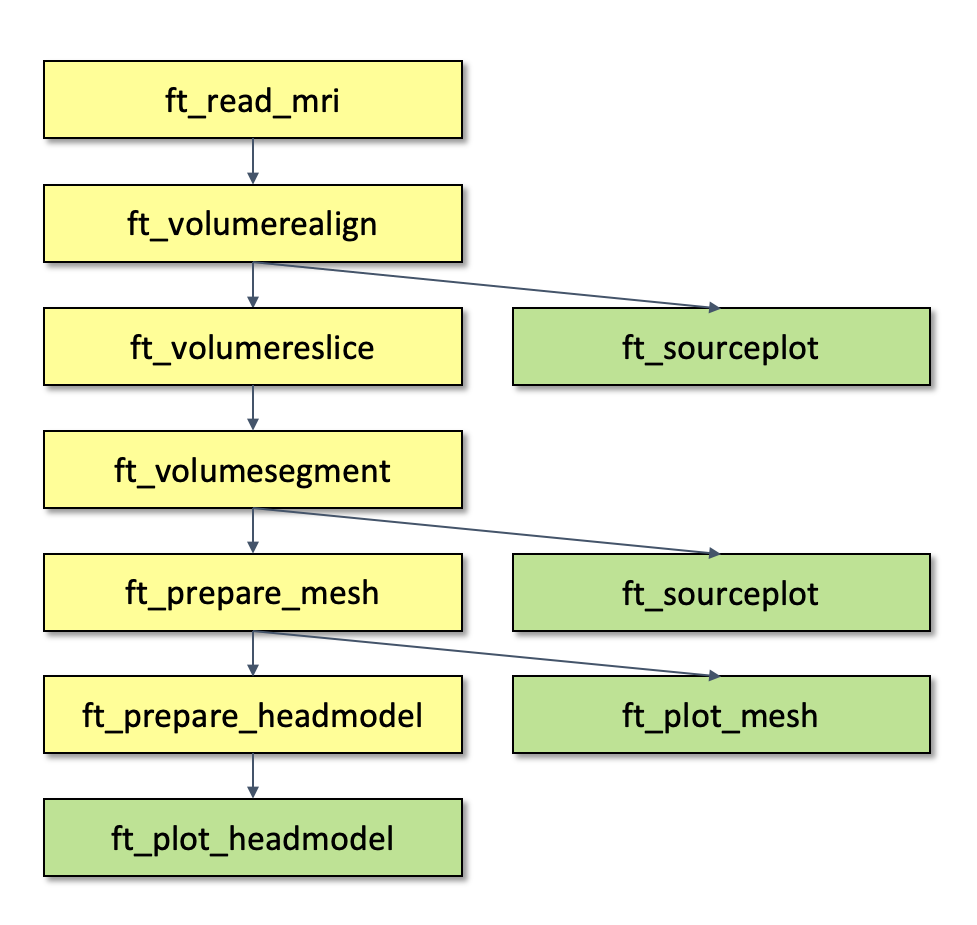Figure 2. Pipeline of creating a BEM model

## Reading in the anatomical data

Before starting with FieldTrip, it is important that you set up your MATLAB path properly.

``````cd PATH_TO_FIELDTRIP
ft_defaults
``````

Then, you can read in the mri data.

``````mri = ft_read_mri('Subject01.mri');

disp(mri)
dim: [256 256 256]
anatomy: [256x256x256 int16]
hdr: [1x1 struct]
transform: [4x4 double]
coordsys: 'ctf'
``````

The structure of your mri variable contains the following field

• dim: This field gives information on the size (i.e. the number of voxels) of the anatomical volume into each direction.
• anatomy: This is a matrix (with the size and number of dimensions specified in dim) that contains the anatomical information represented by numbers.
• hdr: Header information of the anatomical images.
• transform: A homogenous transformation matrix that aligns the anatomical data (in field anatomy) to a certain coordinate system.
• coordsys: The description of the coordinate system which the anatomical data is aligned to.

You can see that the coordsys field of anatomical data that we read in is already aligned to the ctf coordinate system.

Later in this tutorial, we will segment the anatomical MRI. Segmentation works properly when the voxels of the anatomical images are homogenous (i.e. the size of the voxel is the same into each direction). If you do not have homogenous voxels (or you are not sure of), you can use the ft_volumereslice function on the anatomical data before segmentation. Read more about re-slicing here.

## Align MRI to the head coordinate system

When you prepare a head model for EEG, the head model should be in the same coordinate system as the electrodes. It is not relevant in which coordinate system the geometrical information is defined, until all are aligned. For this, you can do two thing

• either you need to align the anatomical MRI (before the segmentation) into the same coordinate system in which the electrodes will be expressed. For example, if you want to align the anatomical MRI to the ctf coordinate system, it can be aligned with using the ft_volumerealign function. For this alignment, you will need to align your MRI to the fiducial points (LPA, RPA and nasion). The output of any later processing step (segmentation, mesh, headmodel) will be expressed in the same coordinate system as your anatomical mri. And then, you can also align the electrodes to the same points.

• or you can also align later your electrodes interactively or manually to an existing head model.

The anatomical MRI that we use in this tutorial is already aligned to a head coordinate system (ctf). We also have information (see later) how the EEG electrodes are positioned relative to the fiducials. Therefore, we do not need to align the anatomical MRI to any other convention.

It is also possible to read in anatomical MRI data in other formats, which are defined in a different coordinate system. When you read in your own anatomical data, it may does not give information on the coordinate system in which the anatomical data is expressed and/or maybe there is no transformation matrix specified. In this case, you can check the coordinate-system with the ft_determine_coordsys function.

## Segmentation

In this step, the voxels of the anatomical MRI are segmented (i.e. separated) into the three different tissue types: scalp, skull and brain. The function ft_volumesegment will produce the required output. You can read more about how the tissue-types are represented in the output of this function in this FAQ.

Note that the segmentation is quite time consuming (~15mins) and if you want you can load the result and skip ahead to the next step. You can download segmentedmri.mat which contains the segmented MRI used in this tutorial.

Occasionally, the quality of the anatomical image is not sufficient to provide a good segmentation out-of-the-box. This could for example happen if the anatomical MRI has been acquired with the subject wearing an EEG cap. This could cause image inhomogeneities that make the automatic segmentation fail. One possible solution could be to correct for these inhomogeneitie using the ft_volumebiascorrect function. For more information, you can consult this frequently asked question.

``````cfg           = [];
cfg.output    = {'brain','skull','scalp'};
segmentedmri  = ft_volumesegment(cfg, mri);

save segmentedmri segmentedmri

disp(segmentedmri)
dim: [256 256 256]
transform: [4x4 double]
coordsys: 'ctf'
unit: 'mm'
brain: [256x256x256 logical]
skull: [256x256x256 logical]
scalp: [256x256x256 logical]
cfg: [1x1 struct]
``````

The segmentedmri data structure is similar to the mri data structure, but contains the new field

• unit: unit of the head coordinate system
• brain: binary representation of the brain
• skull: binary representation of the skull
• scalp: binary representation of the scalp
• cfg: configuration information of the function which created segmentedmri

The segmentation does not change the coordinate system, nor the size of the volume. You can see this in the first three fields (dim, transform and coordsys) which are the same as the corresponding fields of the input mri data structure. But now, the field transform aligns the matrix in field brain, skull and scalp to the coordinate system defined in the coordsys field. It is good practice to check at this point in a figure, whether the segmented compartments look as expected.

## Mesh

In this step, surfaces are created at the borders of the different tissue-types by the ft_prepare_mesh function. The output of this function are surfaces which are represented by points (vertices) connected in a triangular way. The tissues from which the surfaces are created have to be specified and also the number of vertices for each tissue.

``````cfg=[];
cfg.tissue={'brain','skull','scalp'};
cfg.numvertices = [3000 2000 1000];
bnd=ft_prepare_mesh(cfg,segmentedmri);

save bnd bnd

disp(bnd(1))
pnt: [3000x3 double]
tri: [5996x3 double]
unit: 'mm'
``````

The bnd contains the following field

• pnt : The coordinates of the vertices of the surface.
• tri : Each row defines three vertices (row numbers of the pnt field) from a triangle.
• unit: Units in which the points are expressed.

It is a structure array which describes the geometry of three surfaces in these fields. The first structures represents the triangulation of the brain surface, the second the outside surface of the skull and so on.

The scalp, skull and brain mask have already been segmented and a surface description of the brain has been constructed. Now, we will create the volume conduction model. We will specify method ‘dipoli’, and ‘openmeeg’ to build the head model in the cfg.method field, but there also other methods to build a BEM model. For example, method ‘dipoli’ will not work on a Windows platform. In this case, you can either use ‘openmeeg’, ‘bemcp’, or another method or you can download the headmodel.

``````% Create a volume conduction model using 'dipoli', 'openmeeg', or 'bemcp'.
% Dipoli
cfg        = [];
cfg.method ='dipoli'; % You can also specify 'openmeeg', 'bemcp', or another method.

bnd: [1x3 struct]
cond: [0.3300 0.0041 0.3300]
mat: [6000x6000 double]
type: 'dipoli'
unit: 'mm'
cfg: [1x1 struct]
``````

The headmodel data structure contains the following fields:

• bnd: contains the geometrical description of the head model.
• cond: conductivity of each surface
• mat: matrix
• type: describes the method that was used to create the headmodel.
• unit: the unit of measurement of the geometrical data in the bnd field
• cfg: configuration of the function that was used to create the headmodel

The bnd field contains the same information as the mesh we created in the earlier step. But the headmodel also contains a conductivity value for each surface and a matrix used for the volume conduction model. Note that the unit of measurement used in the geometrical description of headmodel is in ‘mm’. The EEG sensors should be also defined in ‘mm’. The units of all type of geometrical information should be the same when a leadfield is computed for source-reconstruction.

The order in which different tissue types are represented in the output of ft_prepare_headmodel may depend on the volume conduction model you are using. Make sure to double-check which tissue type is represented where in headmodel.bnd.

## Visualization

The headmodel contains three structures in the bnd field. These are the geometrical descriptions of the scalp, skull and brain surfaces. First, we will plot each of the surfaces using the ft_plot_mesh function. Then, all surfaces will be plot together on the same figure.

``````figure;
figure;
figure;
``````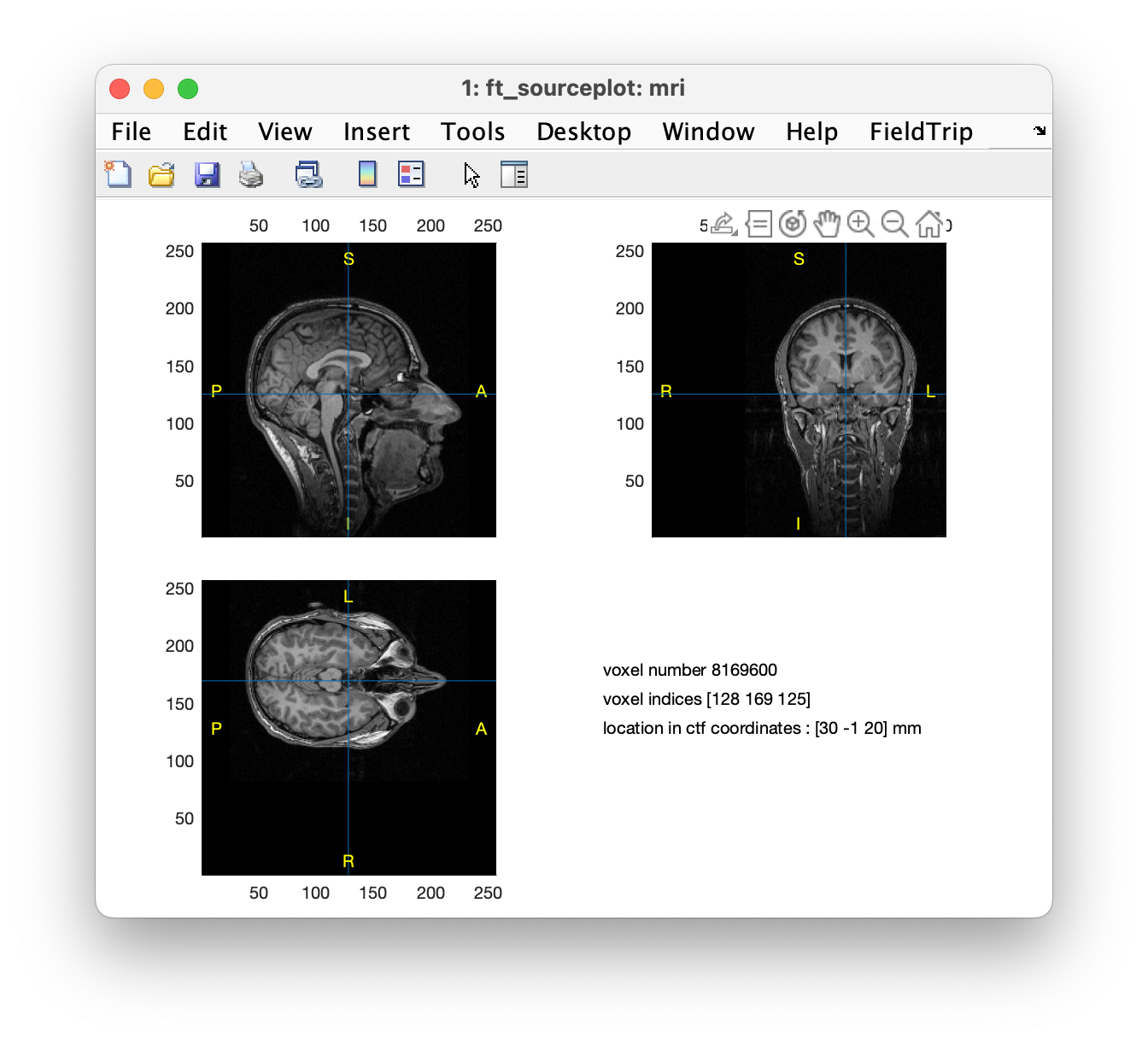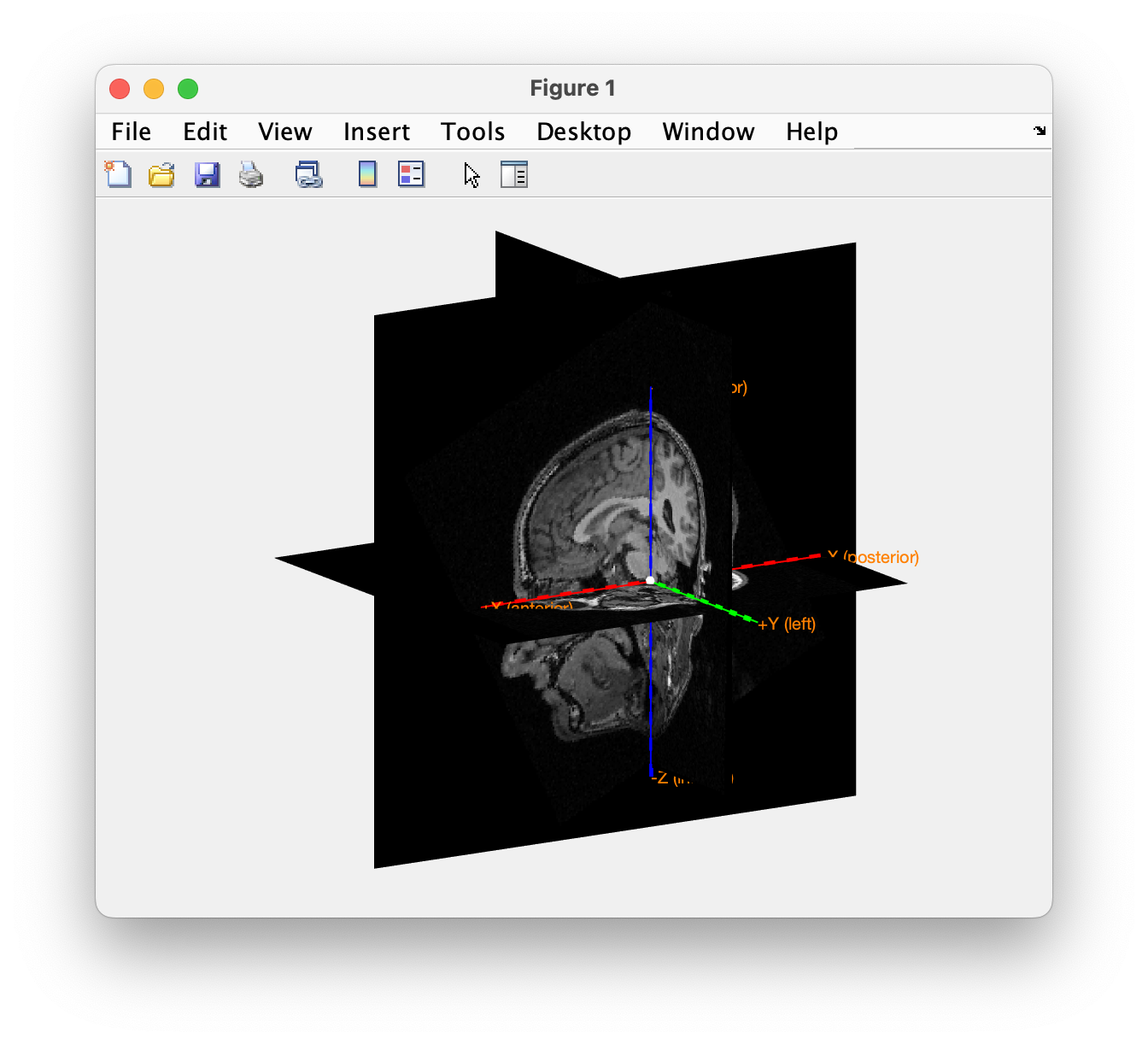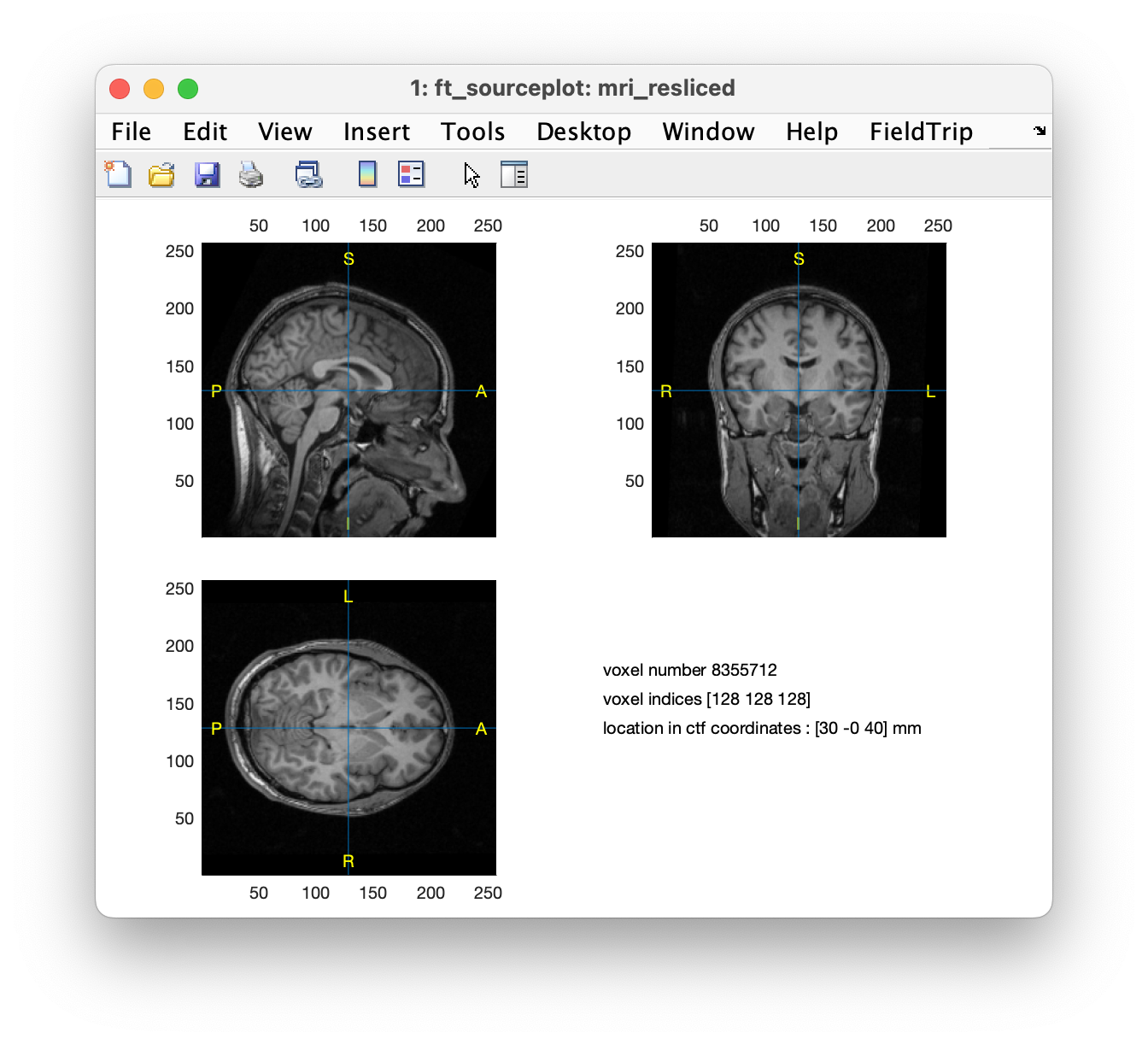Figure 3. The geometry of the volume conduction model using BEM (‘dipoli’): scalp (left), skull (middle) and brain (right)

``````ft_plot_mesh(headmodel.bnd(1), 'facecolor',[0.2 0.2 0.2], 'facealpha', 0.3, 'edgecolor', [1 1 1], 'edgealpha', 0.05);
hold on;
hold on;
``````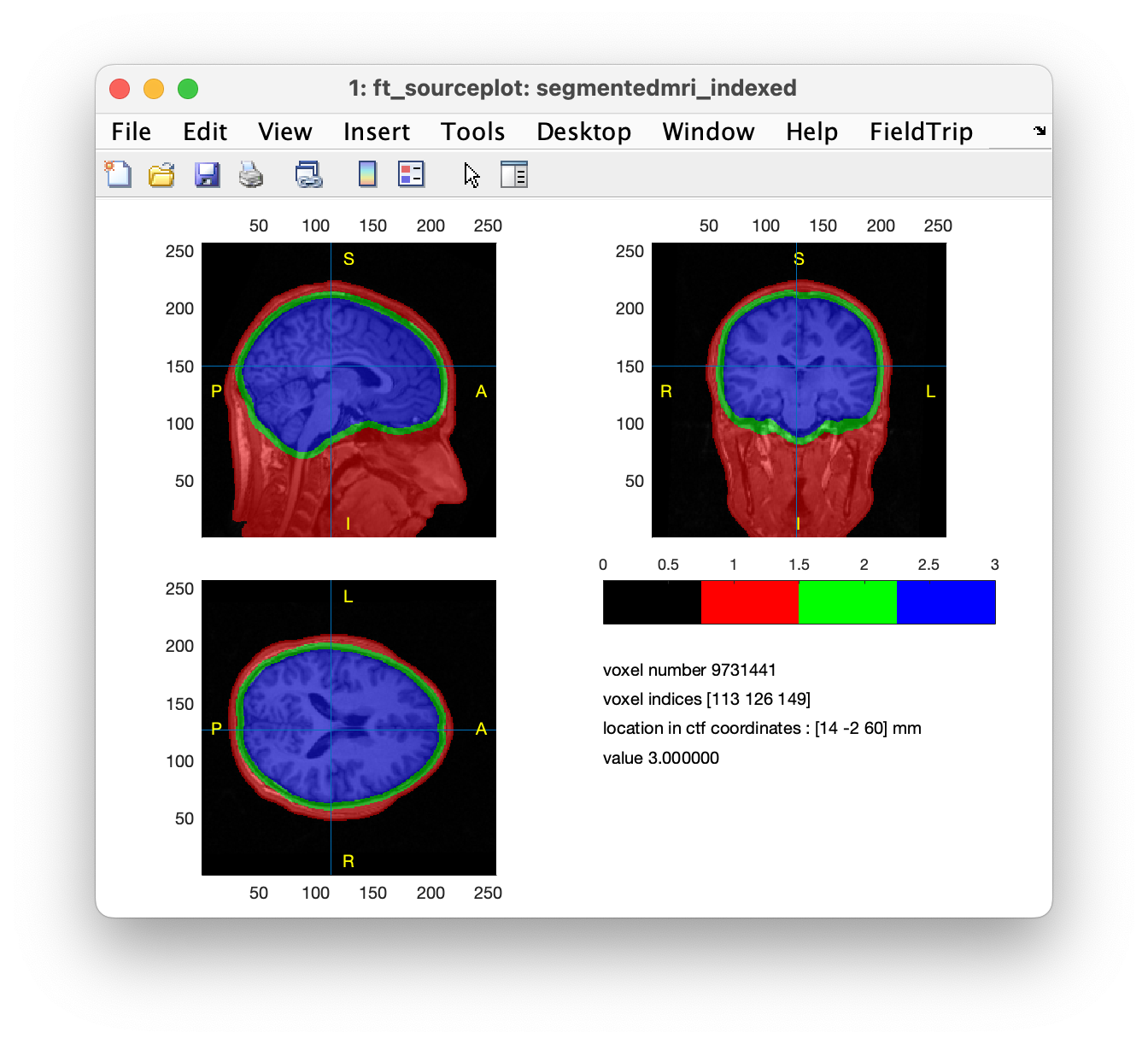Figure 4. The geometry of the volume conduction model. All surfaces (scalp:gray,skull:white,brain:green) plotted together

When the figure is plotted, you can look at the figure from different views using the curved arrow in the MATLAB figure menu.

## Align the electrodes

The head model is expressed in head coordinates of the anatomical mri (ctf coordinate system). We need to define the electrode positions in the same head coordinate system. First, we plot the outermost layer of the head model (scalp) together with the electrodes to check if the alignment is necessary. We use a template set of electrodes which you can find in the FieldTrip/template/electrode/standard_1020.elc file.

``````% you may need to specify the full path to the file

disp(elec)
chanpos: [97x3 double]
elecpos: [97x3 double]
label: {97x1 cell}
type: 'ext1020'
unit: 'mm'
``````

The electrode positions are described in the elecpos field. The label field contains the name of the electrodes.

``````% load volume conduction model
figure;
% head surface (scalp)
ft_plot_mesh(headmodel.bnd(1), 'edgecolor','none','facealpha',0.8,'facecolor',[0.6 0.6 0.8]);
hold on;
% electrodes
ft_plot_sens(elec,'style', 'sk');
``````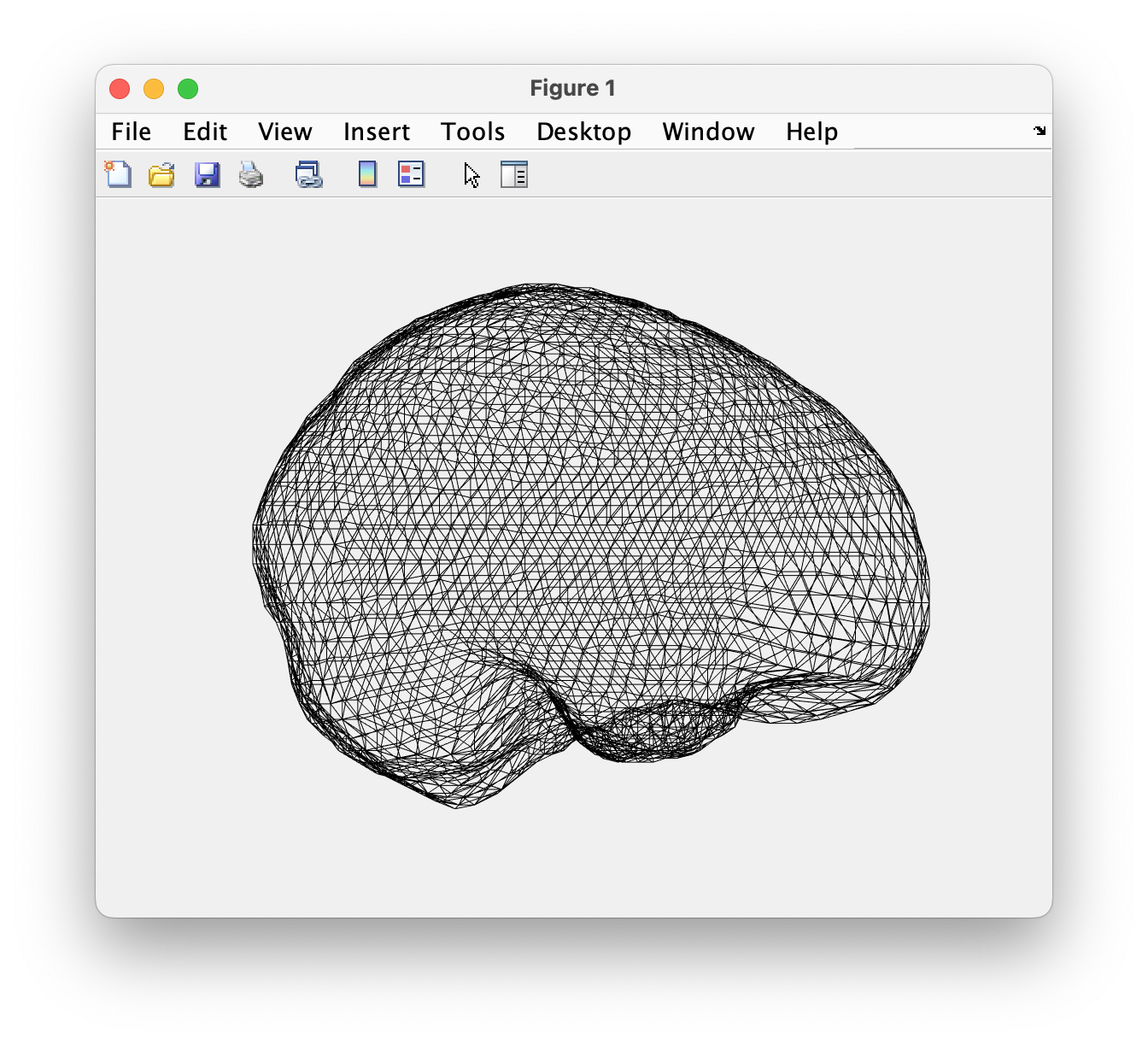Figure 5.

The figure shows that the electrodes are not aligned with the scalp surface.

The electrodes can be aligned in two way

• if there are anatomical landmarks which positions are known in the anatomical mri and also relative to the electrodes, we can automatically align the electrode positions with a few lines of script or

• if the exact position of anatomical landmarks are not known relative to the electrodes, we visualize the surface of the head and the electrodes on the same image, and we transform, rotate and scale the electrodes until they fit to the head surface according to our visual judgement.

Now, we will show how to do the alignment in both way

### Automatic alignment

First, we will align the electrodes automatically to the anatomical landmarks of the anatomical mri. The head model was created from the same mri, therefore the electrodes will also be aligned to the head-model.

For the automatic alignment, we need three pieces of informatio

• electrode positions

• position of fiducial landmarks relative to the electrodes

• position of fiducial landmarks in the anatomical mri

In the template set of electrodes, the first three labels are: ‘Nz’, ‘LPA’ and ‘RPA’. These labels show that the first three rows of the elec.chanpos field defines the position of the nasion, left and right PA (the landmarks of the CTF ) in “electrode” coordinates. We can use this information for the automatic alignment. But we also need to know the position of the same points in the anatomical mri. We use an anatomical mri which has been already aligned to these points, therefore we can find these coordinates in the header information.

``````mri = ft_read_mri('Subject01.mri');

disp(mri.hdr.fiducial.mri)
nas: [87 60 116]
lpa: [29 145 155]
rpa: [144 142 158]
``````

If you do not have the position of the anatomical landmarks in your volume, you can use the ft_volumerealign function to get those positions.

First, we get these positions in the ctf coordinate system using the transformation matrix of the mri and the ft_warp_apply function.

``````nas=mri.hdr.fiducial.mri.nas;
lpa=mri.hdr.fiducial.mri.lpa;
rpa=mri.hdr.fiducial.mri.rpa;

transm=mri.transform;

nas=ft_warp_apply(transm,nas, 'homogenous');
lpa=ft_warp_apply(transm,lpa, 'homogenous');
rpa=ft_warp_apply(transm,rpa, 'homogenous');
``````

Then, we align the position of the fiducials in the electrode structure (defined with labels ‘Nz’, ‘LPA’, ‘RPA’) to their ctf-coordinates that we acquired from the anatomical mri (nas, lpa, rpa).

``````% create a structure similar to a template set of electrodes
fid.elecpos       = [nas; lpa; rpa];       % ctf-coordinates of fiducials
fid.label         = {'Nz','LPA','RPA'};    % same labels as in elec
fid.unit          = 'mm';                  % same units as mri

% alignment
cfg               = [];
cfg.method        = 'fiducial';
cfg.target        = fid;                   % see above
cfg.elec          = elec;
cfg.fiducial      = {'Nz', 'LPA', 'RPA'};  % labels of fiducials in fid and in elec
elec_aligned      = ft_electroderealign(cfg);

save elec_aligned elec_aligned;
``````

We can check the alignment by plotting together the scalp surface with the electrodes.

``````figure;
ft_plot_sens(elec_aligned,'style','sk');
hold on;
ft_plot_mesh(headmodel.bnd(1),'facealpha', 0.85, 'edgecolor', 'none', 'facecolor', [0.65 0.65 0.65]); %scalp
``````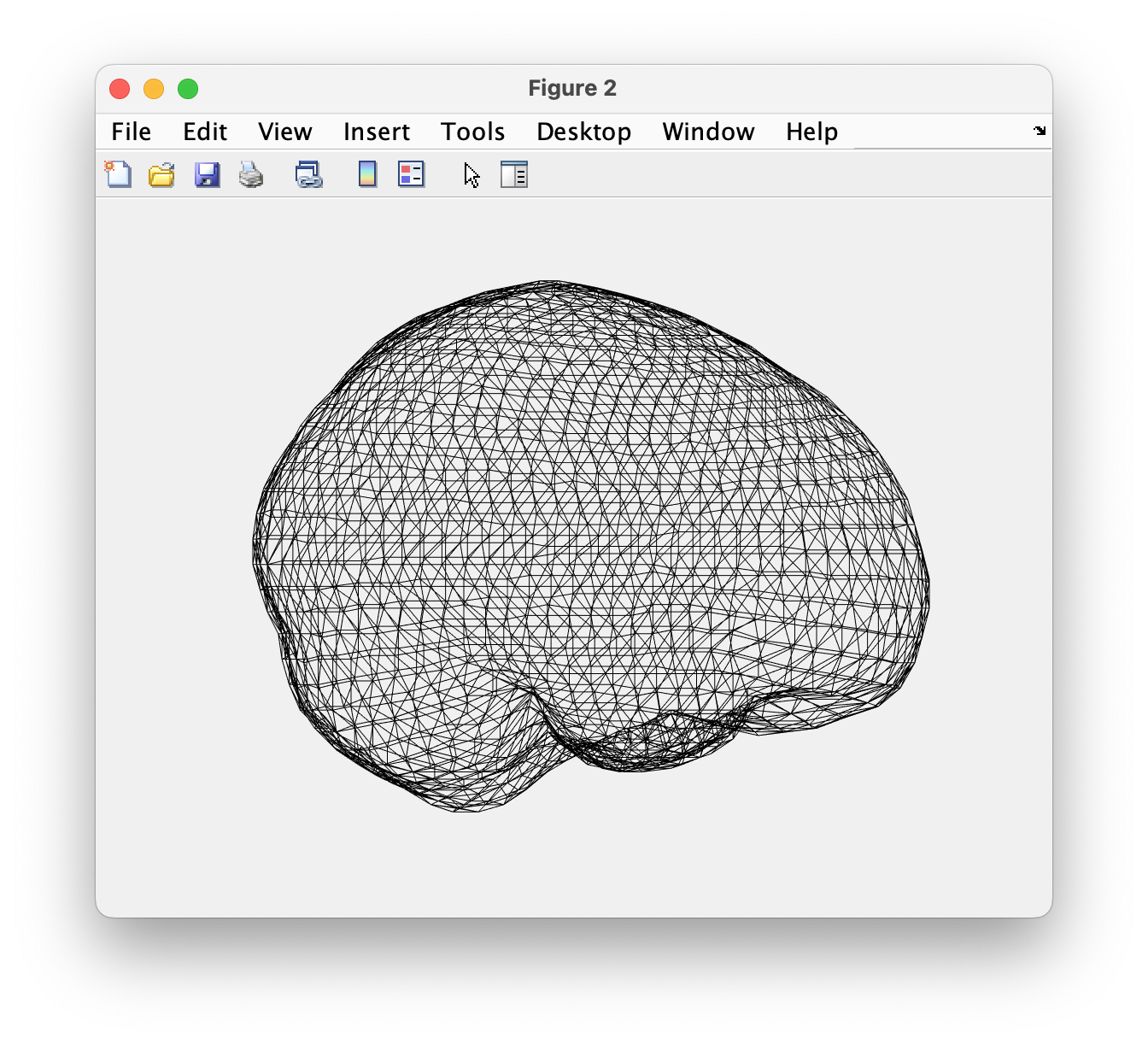Figure 6. Electrodes plotted together with the scalp surface.

Some of the electrodes are below the skin in the front, while the electrodes in the back do not fit tightly to the head. This happened because there are different conventions to define the fiducials. < he anatomical MRI is in CTF coordinates with the fiducials for the left and right ear aligned with the ear canal according to DCCN convention.

The description of the electrodes includes the position of the left and right pre-auriciular point proper, i.e. the point of the posterior root of the zygomatic arch lying immediately in front of the upper end of the tragus.

One way to fix the misalignment is to provide the location of consistent fiducial locations. In this case it could be implemented by specifying the LPA and RPA point in the anatomical MRI shifted approximately 20 mm more anterior.

In the subsequent section however, we try to improve the alignment of the electrodes interactively.

### Interactive alignment

``````cfg           = [];
cfg.method    = 'interactive';
cfg.elec      = elec_aligned;
elec_aligned  = ft_electroderealign(cfg);
``````

Here, we only need to use translation. We can shift the x axis with a few mm (12). This will move the electrodes more towards the front of the head. (Note: the positive x is towards the nasion in the ctf ccordinate system.) The electrodes fit better to the head surface after the translation.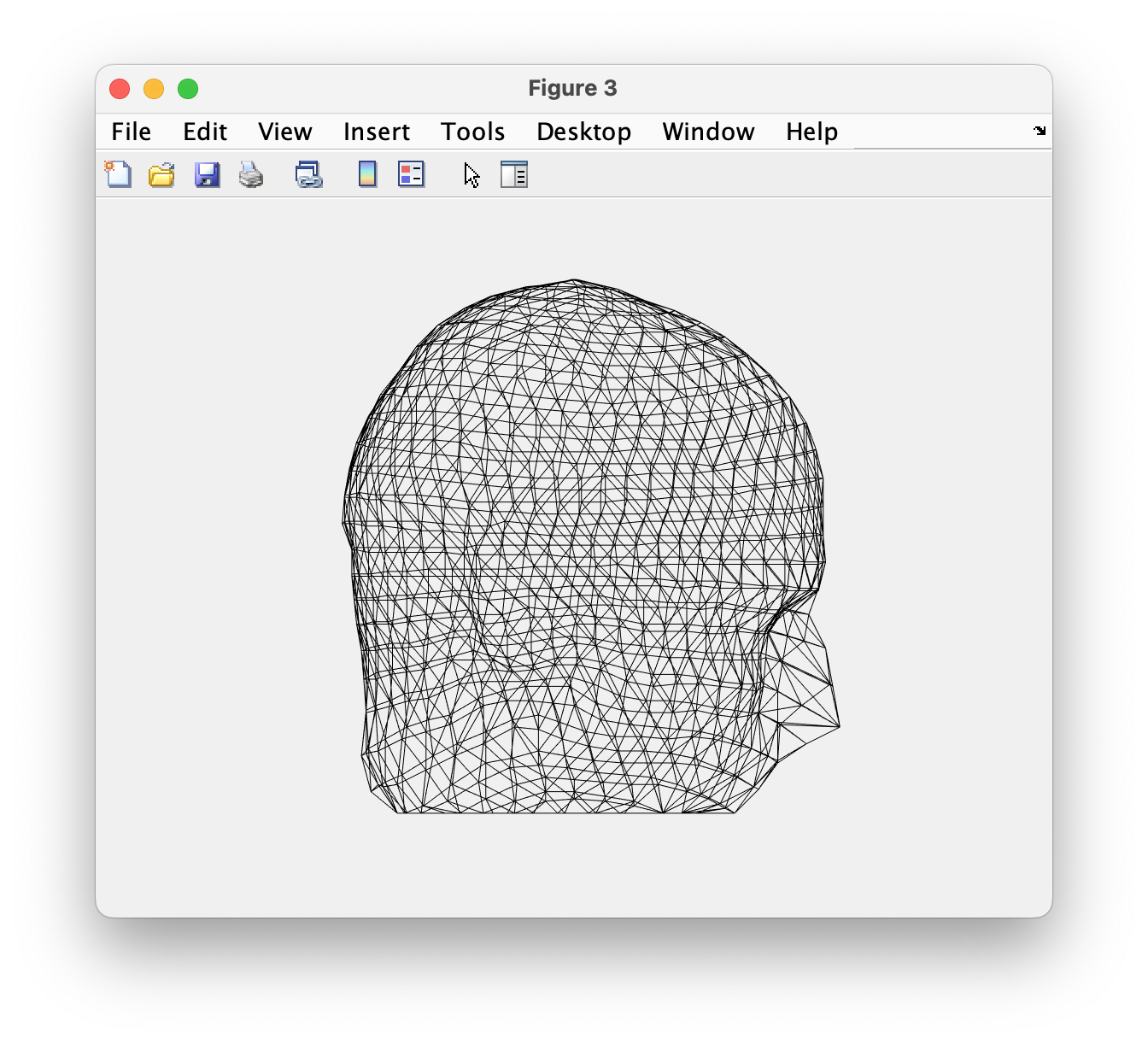Figure 7. Aligned electrodes plotted together with the scalp surface

This electrode structure can be used later when the leadfield is computed during source-reconstruction. During the computation of the leadfield, the electrodes will be projected onto the scalp surface.

## Exercise 1

Create a head model with method ‘concentricspheres’ that you fit on scalp, skull and brain surfaces, i.e. using the already made mesh.

Plot the head model in the same figure with the brain surface and scalp. Check the help of ft_plot_headmodel for further options of the visualization (e.g., color, transparency) which help to see the spheres and the brain surface together.

What is the difference between this head model and the BEM?

## Exercise 2

In exercise 1, you created a head model with method ‘concentricspheres’. How is its geometrical description defined? What is the difference between the geometrical description of the concentric spheres model and BEM model?

## Summary and further reading

In this tutorial, it was explained how to build a volume conduction model of the head using a single subject anatomical mri and the boundary element method (BEM) developed by Oostendorp and van Oosterom (1989). In the exercises, we compared the BEM model to a concentric spheres model that was fitted on the scalp, skull and brain surfaces.

You can read more about specific source-reconstruction methods in the Localizing oscillatory sources using beamformer techniques and in the Source reconstruction of event-related fields using minimum-norm estimate tutorials.

Here are the related FAQs:

and the related examples: